﻿ 螺旋桨脉动压力及噪声特性的数值模拟研究
 舰船科学技术2016, Vol. 38Issue (8): 34-38PDF

Numerical investigation of near-field fluctuating pressure and far-field noise characteristic induced by a propeller
WANG Li, SUN Guo-cang, XU Jun
Wuhan Second Ship Design and Research Institute, Wuhan 430205, China
Abstract: In this paper, the near-field fluctuating pressure and far-field noise induced by a propeller is investigated with LES and FW-H method. Analysis of fluctuating pressure in near field reveal that, the frequency performace of fluctuating pressure in the disk-direction is different from that in the axis-direction. The amplitude of fluctuating pressure decreases rapidly with increasing distance. It is also found that the distance between sound pressure receiver and noise source must be longer than 10 times of propeller diameter to avoid the influence of pseudo-sound. Analysis of noise directivity in the far field reveals that, the total sound pressure level is uniform distribution in the disk plane, while that is elliptical distribution in the longitudinal plane. It is also found that the frequency characterisc of sound radiation in far filed is directivity.
Key words: propeller     fluctuating pressure     noise directivity     frequency characteristic
0 引言

1 数值方法 1.1 计算方法

Lighthill将流体运动发声的复杂现象用静态介质中存在等效声源的声学问题来进行类比，认为流噪声是由速度波动、熵波动、粘性应力的非线性相互作用而产生的流动非稳定引起。Lighthill方程为：

 $c_0^2{\nabla ^2}{\rho ^{'}}-\frac{{{\partial ^2}{\rho ^{'}}}}{{{\partial ^2}{t^2}}} = -\frac{{\partial Q}}{{\partial t}} + \nabla \cdot \vec F-\frac{{{\partial ^2}{T_{ij}}}}{{\partial {x_i}\partial {y_j}}}\text{，}$ (1)
 ${T_{ij}} = \rho {U_i}{U_j} + {\delta _{ij}}(p-c_0^2{\rho ^{'}})-{\tau _{ij}}\text{。}$ (2)

 $c_0^2{\nabla ^2}{\rho ^{'}}-\frac{{{\partial ^2}{\rho ^{'}}}}{{{\partial ^2}{t^2}}} = -\left[{\frac{{\partial Q}}{{\partial t}}-\nabla \cdot \vec F + \frac{{{\partial ^2}({\rho _0}{U_i}{U_j})}}{{\partial {x_i}{x_j}}}} \right]\text{。}$ (3)

Lighthill方程为自由空间假设下得到，FW-H方程考虑了流体中包含任意运动表面的情况：

 $\begin{array}{l} c_0^2{{\bar \nabla }^2}{\rho ^{'}}-\displaystyle\frac{{{{\bar \partial }^2}{\rho ^{'}}}}{{{\partial ^2}{t^2}}} = -\displaystyle\frac{{\bar \partial }}{{\partial t}}\left\{ {{\rho _0}{\nu _n}\left| {\nabla f} \right|\delta (f)} \right\} + \\[10pt] \quad \quad \quad \quad \!\!\displaystyle\frac{{\bar \partial }}{{\partial {x_i}}}\left\{ {{L_i}\left| {\nabla f} \right|\delta (f)} \right\}-\frac{{{{\bar \partial }^2}{T_{ij}}}}{{\partial {x_i}\partial {x_j}}}\text{，} \end{array}$ (4)
 ${L_i} = {p{'}_{ij}} \cdot {n_j} = {p{'}_{ij}}\frac{{\partial f/\partial {x_j}}}{{\left| {\nabla f} \right|}}\text{。}$ (5)

FW-H方程包含以下3部分噪声源：分布在表面以外的密度为Tij的四极子源；分布在表面上密度为Li的偶极子源；分布在表面上密度为ρ0νn的单极子源。

1.2 计算对象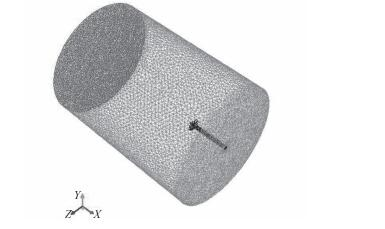图 1 螺旋桨计算域示意图 Fig. 1 Schematic of the propeller computational domain
1.3 计算参数

2 计算结果及分析 2.1 近场脉动压力频率特性分析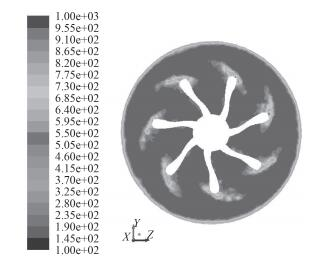图 2 桨盘面脉动压力均方根分布云图 Fig. 2 RMS of fluctuating pressure in the disk plane表 1 近场监测点位置 Tab.1 Location of near-field fluctuating pressure monitor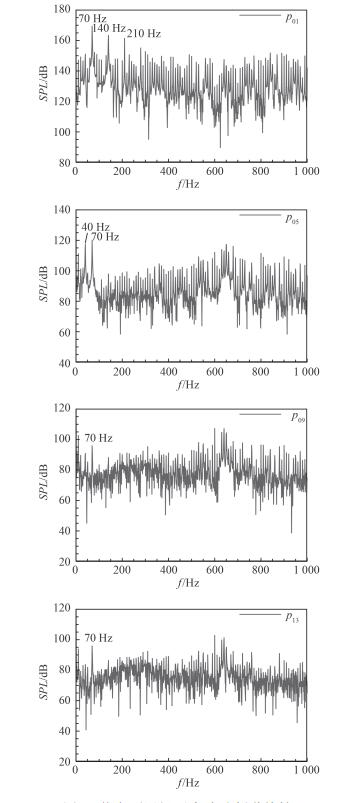图 3 桨盘面近场压力脉动频谱特性 Fig. 3 Frequency characteristic of near-field fluctuating pressure in the disk plane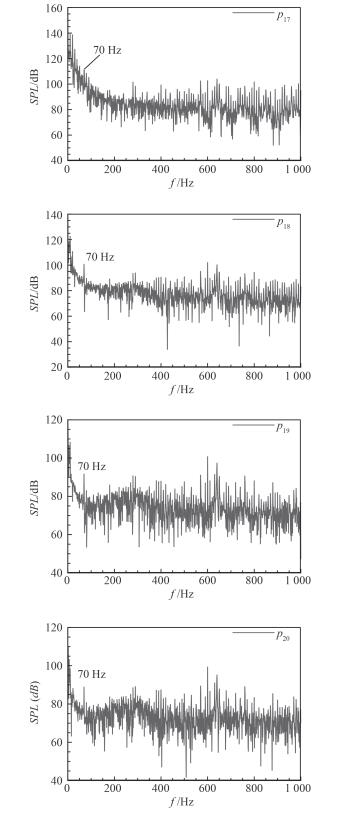图 4 桨轴方向近场压力脉动频谱特性 Fig. 4 Frequency characteristic of near-field fluctuating pressure in the axis plane
2.2 近场脉动压力衰减特性分析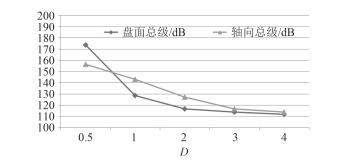图 5 脉动压力总级沿盘面径向和轴向分布 Fig. 5 Distribution of total fluctuating pressure in the radial and axis direction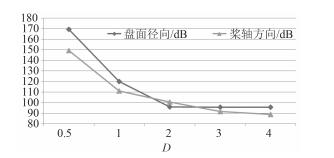图 6 脉动压力一阶叶频幅值沿盘面径向和轴向分布 Fig. 6 Distribution of BPF fluctuating pressure in the radial and axis direction
2.3 噪声衰减特性分析

 ${\left( {SPL} \right)_r} = {\left( {SPL} \right)_{100 {\rm m}}}{\text{-}}20\log \left( {r/100} \right)\text{。}$ (6)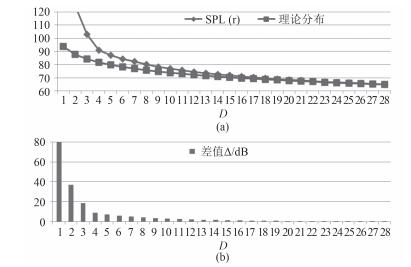图 7 总声级随距离的变化情况 Fig. 7 Distribution of total sound pressure level with different distance
2.4 远场噪声指向性分析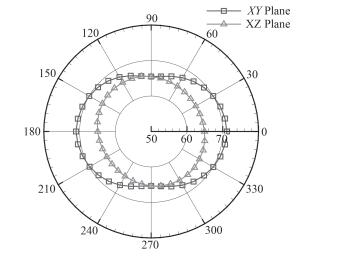图 8 桨盘面和纵向平面噪声指向性分布 Fig. 8 Directivity of noise in disk plane and axis plane
2.5 远场噪声频谱特性分析表 2 远场声压接收点位置 Tab.2 Location of far fieled sound pressure receiver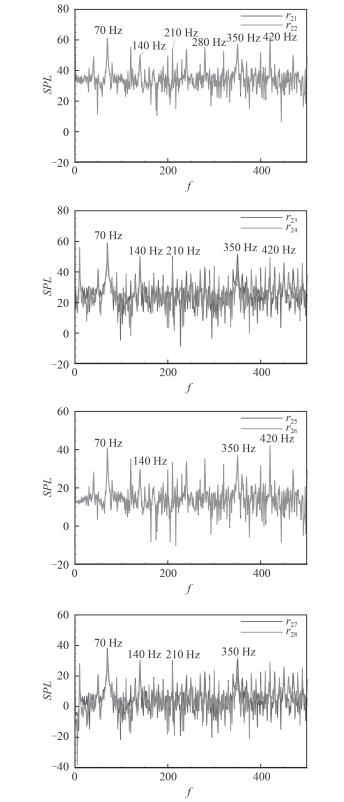图 9 远场声压频谱分布 Fig. 9 Distribution of far field sound pressure level with frequency
3 结语

1）螺旋桨近场脉动压力频率特性在桨盘面和桨轴方向存在差异，桨盘面叶频线谱突出，桨轴方向主要由轴频线谱占主导，随着距离增大，脉动压力迅速衰减；采用Fluent中FW-H方法计算辐射噪声时，声场接收点距声源距离必须大于10倍的桨叶直径。

2）螺旋桨远场声辐射的指向性分析表明，噪声总级在桨盘面轴向均匀分布，在纵向平面呈现椭圆形分布，桨轴方向声压比桨盘面声压大。进一步分析远场声辐射的频率特性发现，桨盘面声辐射轴频线谱突出，而桨轴方向声辐射轴频线谱不明显。

  汤渭霖. 螺旋桨涡旋噪声预报[J]. 船舶力学 , 1999, 3 (2) :49–57.  朱锡清, 吴武生. 螺旋桨负荷噪声研究[J]. 声学学报 , 1999, 24 (3) :259–268.  朱锡清, 唐登海, 孙红星, 等. 船舶螺旋桨低频噪声研究[J]. 船舶力学 , 2000, 4 (1) :50–55.  张永坤, 熊鹰, 赵小龙. 螺旋桨无空泡噪声预报[J]. 噪声振动与控制 , 2008, 28 (1) :44–47.  王超.螺旋桨水动力性能、空泡及噪声性能的数值预报研究[D].哈尔滨:哈尔滨工程大学, 2010. http://www.oalib.com/references/18936664  谢剑波, 周其斗. 非均匀流场中螺旋桨线谱噪声指向性分析[J]. 中国舰船研究 , 2010, 5 (6) :6–11.  杨琼方, 王永生, 曾文德, 等. 大侧斜螺旋桨负载噪声的边界元数值声学方法频域内计算分析[J]. 兵工学报 , 2011, 32 (9) :1118–1125.  张漫.螺旋桨无空泡噪声的数值预报研究[D].大连:大连理工大学, 2013. http://cdmd.cnki.com.cn/article/cdmd-10141-1013198835.htm  SEOL H, JUNG B, SUH J C, et al. Prediction of non-cavitating underwater propeller noise[J]. Journal of Sound and Vibration , 2002, 257 (1) :131–156. DOI:10.1006/jsvi.2002.5035  SEOL H, SUH J C, LEE S. Development of hybrid method for the prediction of underwater propeller noise[J]. Journal of Sound and Vibration , 2005, 288 (1/2) :345–360.  SCHRAM C, MARTÍNEZ-LERA P, TOURNOUR M. Two-dimensional in-duct vortex leapfrogging as a validation benchmark for internal aeroacoustics[C]//Proceedings of the 13th AIAA/CEAS aeroacoustics conference (28th AIAA Aeroacoustics Conference). Rome, Italy: AIAA, 2007.